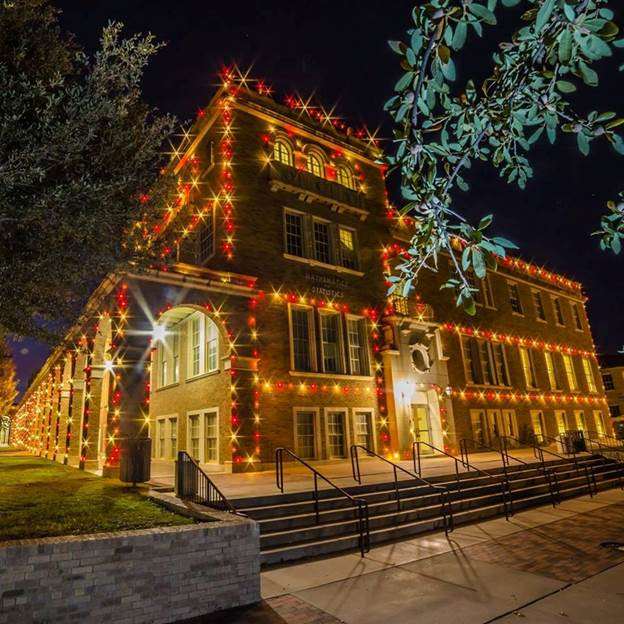﻿ Dr. Magdalena Toda, ChairProfessor Magdalena Toda
My main research interests are in differential geometry and related integrable systems, and geometric applications to the following fields: molecular biology, geometry of biological membranes, fluid flow in physics and engineering, 2D and 3D geometric image analysis. My dissertation (2000) and early works emphasized certain applications of moving frame methods and Lie group theory to specific surface constructions. My main research area has been in pure Mathematics (Riemannian spaces, relativistic spaces, geometric solutions of PDE), but I have devoted a large part of my research time to applications. Since 2009, I have developed a great interest in the differential geometry of integrable systems that occur in Physics and Engineering (nonlinear fluid flows), as well as Microbiology and Biophysics (the geometry of protein folding, beta sheets and beta barrels, geometric evolution) in conjunction with generalized Willmore type surface and their flows.

Between 1998 and 2000, I completed my PhD dissertation on a generalized Lie-algebraic representation (similar to the Weierstrass representation for minimal surfaces) for the pseudospherical surfaces (constant negative Gauss curvature surfaces) in Euclidean space. This problem appears from Geometry and PDEs all together, as related to the Goursat problem for the sine-Gordon equation. A modern loop group factorization tool allowed the construction of these surfaces, a technique that was numerically implemented by N. Schmidt and I. Sterling and allowed unprecedented visualization of pseudospherical surfaces and their generalized-Backlund transformations. Further, I have become increasingly interested in harmonic maps. I studied harmonic map equations, not just as partial differential equations per se, but as conditions for certain loops of connections to verify the zero-curvature equation. I have studied the various Weierstrass-type representations of Weingarten surfaces. At the same time, I had completed a PhD in Applied Mathematics at the University Politehnica Bucharest, with an emphasis on Riemannian manifold structures associated with electromagnetic fields.

Between 2000 and 2003, my collaborators (J. Dorfmeister and I. Inoguchi) and I derived corresponding Lie-algebraic representations for minimal timelike and CMC timelike surfaces in Lorenz 3-space. I have learned a lot about general surface representations, especially those of type Weierstrass (e.g., Weierstrass-Kenmotsu-Bryant) and, between 2003 and 2005, I have extended some of these results to CMC surfaces in hyperbolic space.

Since 2007, I have worked in Geometry and PDE (integrable systems) and its real-world applications. A joint work with R. Iyer on an optical navigational system based on the geometry of the flight of insects had completely changed my perspective on Differential Geometry: it gave me an understanding on the Fundamental Role played by Riemannian geometries and curvature tensors in real-world Mechanics and Physics. I then continued to work on applications of differential geometry, through my joint study with A. Ibragimov and E. Aulisa, on a differentiable equation that is strongly linked to the CMC equation and comes from hydrodynamics. The lattertopic relates to nonlinear fluid flows in petroleum engineering. Under certain assumptions about the fluid, one arrives at a non-linear equation for the pressure function. This equation displays some similarity to the constant mean curvature (CMC) equation for surfaces. We utilized this similarity to find a constraint on the Forchheimer flows, such that the pressure function can be regarded as a surface with constant mean curvature, after a certain geometric transformation. Conversely, a surface with constant mean curvature can be interpreted as a pressure distribution of the flow, subjected to a nonlinear Darcy law similar to the Forchheimer equation. This trade-off between hydrodynamic and geometric problems is not just interesting in itself: moreover, the geometric interpretation of the problem of the flow in porous media gives a simple algorithm for the computation of productivity index of a well, in a structured heterogeneous reservoir.

Since 2009, I have become increasingly interested in molecular biology, in particular protein folding, and its parallels to the theory of elastic membranes. I have proposed new models for beta sheets and beta barrels, a secondary structure that appears in protein folding, based on Willmore-type surfaces. I am currently working in the field of Willmore energy, Willmore surfaces and Willmore flow, as well as their generalizations. This type of highly nonlinear fourth order equation has a strong connection with some real-world elliptic equations of similar type that are studied by my colleagues from the applied mathematics team.

I have also co-authored the newest editions of a Calculus book with M. Strauss and K. Smith. Many students know me primarily as the co-author of that textbook.